Courses

# Industrial Controllers & Compensators - 2

## 20 Questions MCQ Test Topicwise Question Bank for Electrical Engineering | Industrial Controllers & Compensators - 2

Description
This mock test of Industrial Controllers & Compensators - 2 for Electrical Engineering (EE) helps you for every Electrical Engineering (EE) entrance exam. This contains 20 Multiple Choice Questions for Electrical Engineering (EE) Industrial Controllers & Compensators - 2 (mcq) to study with solutions a complete question bank. The solved questions answers in this Industrial Controllers & Compensators - 2 quiz give you a good mix of easy questions and tough questions. Electrical Engineering (EE) students definitely take this Industrial Controllers & Compensators - 2 exercise for a better result in the exam. You can find other Industrial Controllers & Compensators - 2 extra questions, long questions & short questions for Electrical Engineering (EE) on EduRev as well by searching above.
QUESTION: 1

### Assertion (A) : The phase lag network provides an attenuation of 20 log a (a < 1) at high frequencies having transfer function equal to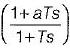Reason (R): The lag network allows to pass high frequencies and low frequencies are attenuated.

Solution:

Here, reason (R) is false because the lag network allows to pass low frequencies and high frequencies are attenuated.

QUESTION: 2

### Assertion (A): Introduction of phase lag network in forward path increases the phase shift. Reason (R): A phase lag network has pole nearer to the imaginary axis as compared to zero.

Solution:

The transfer function of a phase-lag network is: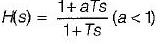The pole-zero plot is shown below: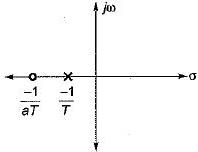Here, pole is nearer to the imaginary axis and origin compared to zero.
Hence, reason (R) is true.
Introduction of phase lag network in forward path reduces the phase shift. Hence, assertion (A) is a false statement.

QUESTION: 3

### Match List-I (Type of controllers) with List-ll (Alternate names) and select the correct answer using the codes given below the lists: List-I A. Two-position controller B. Proportional controller C. Integral controller D. Derivative controller List-II 1. Rate controller 2. Reset controller 3. An amplifier with adjustable gain 4. ON-OFF controller Codes:     A B C D (a) 4 2 3 1 (b) 4 3 2 1 (c) 3 4 2 1 (d) 3 4 1 2

Solution:
QUESTION: 4

The open loop transfer function of a three action controller (PID controller) is given by

Solution:

A PID controller is shown below.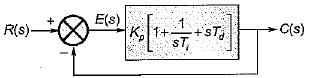Here,
G(s) = O.L.T.F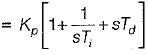QUESTION: 5

The action of a PD controller is to

Solution:

Due to PD controller, a zero is added to the forward path which results in a lead compensator. Therefore, steady state error remais the same whiie rise time of the system is reduced (i.e. stability is increased).

QUESTION: 6

For the derivative feedback control shown in figure below, the damping ratio is equal to 0.7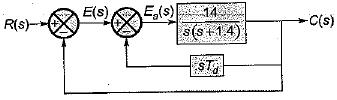The value of derivative gain constant is

Solution:

For the given system, characteristic equation is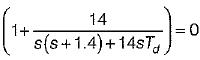or,
s2 + 1.4s + 14s Td + 14 = 0
or,
s2 + (1.4 + 14Td) s + 14 = 0
Comparing with s2 + 2 ξωn + ωn2 =0, we have:
ωn = √14 rad/s and 2ξωn = (1.4 + 14Td)
or, 2 x 0.7 x √14 = 1.4 + 14 Td (∴ ξ = 0.7, given)
or,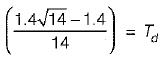or,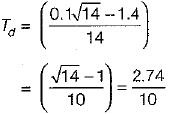= 0.274

QUESTION: 7

The block diagram of an integral control is shown in figure below.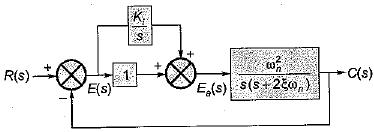The steady state error in the above system for a parabolic input is

Solution:

For the given system, forward path transfer function is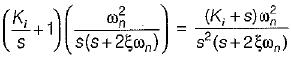For parabolic input, steady state error is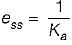where,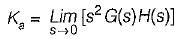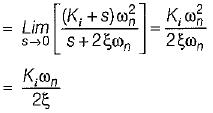∴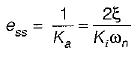QUESTION: 8

A system employing proportional plus error rate control is shown in figure below.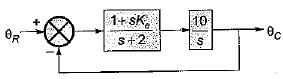The value of error rate control (Ke) and 2% settling time for a damping ratio of 0.5 are respectively

Solution:

The forward path gain of the given system is: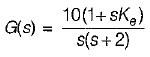∴ Characteristic equation is
1 + G(s)H(s) = 0
or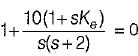or, s2 + (2 + 10 Ke)s +10 = 0
Here,
ω= √10 rad/s
and 2ξω= (2 + 10Ke)
Given, ξ = 0.5
So, 2 x 0.5 x √10 = (2 + 10Ke)
or,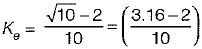= 1.16/10 = 0.116
Also, 2% settling time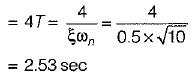QUESTION: 9

A feedback control system employing output- rate damping is shown in figure below.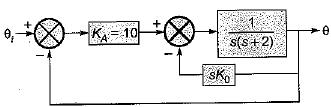What is the steady state error (in radian) for the above system resulting from an unit ramp input if the damping ratio is 0.6?

Solution:

The characteristic equation of the given system is
1+G(s) H(s) = 0
Here,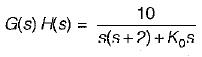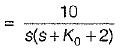∴ Characteristic equation is
10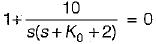or, s2 + (K0 + 2)s +10 = 0
Comparing with s2 + 2ξωns + ωn2 = 0 we have
ωn = √10 rad/s
and 2ξωn = K0 + 2
Given, ξ = 0.6
∴ 2 x 0.6 x √10 = K0 + 2
or, K0 = 1.792
For a unit ramp input, ess = 1/Kv
where,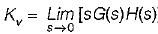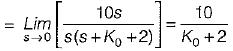∴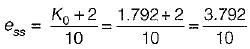= 0.3792 ≈ 0.38 radian

QUESTION: 10

In the control system shown below, the controller which can give infinite value of steady-stae error to a ramp input is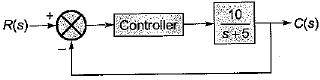Solution:

Case-1: For integral type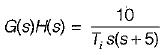∴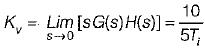∴ ess = Ti/2
Case-2: For derivative type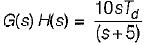G(s)H(s) = (10sTd)/(s+5)
∴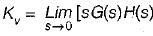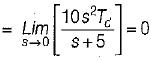∴ ess = 1/Kv =
Case-3: For proportional type
G(s)H(s) = 10Kp/(s+5)
∴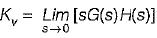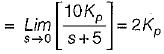∴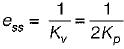Case-4: For proportional plus derivative type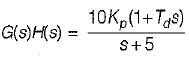∴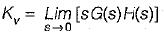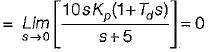∴ ess = 1/Kv = ∞

QUESTION: 11

The transfer function of the OP-AMP circuit shown below is given by: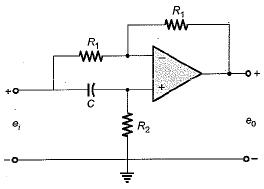Solution:

Redrawing the given circuit in s-domain, we have: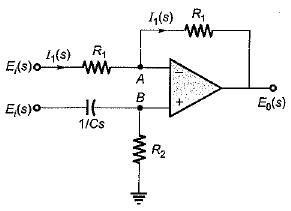Now,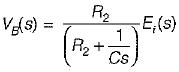From virtual ground concept,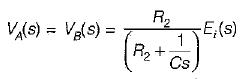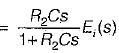Now,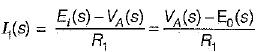or,
2VA(s) = Ei(s) + E0(s)
E0(s) = 2VA(s) - Ei(s)
or,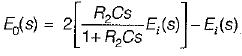or,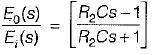QUESTION: 12

The transfer function of a phase lead compensator is given by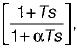where α < 1 and T> 0.
Which of the following is not a correct value of maximum phase shift provided by such a compensator?

Solution:
QUESTION: 13

Assertion (A): Stepping motors are compatible with modern digital systems.
Reason (R): The nature of command is in the forms of pulses.

Solution:

Since the nature of command is in the form of pulses, therefore stepping motors are compatible with modern digital systems.

QUESTION: 14

Consider the control system shown in figure below employing a proportional compensator.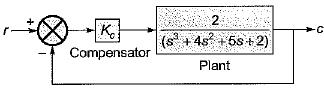If the steady-state error has to be less than two percent for a unit step input, then the value of Kc would be

Solution:

For given system,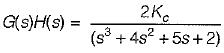With proportional controller,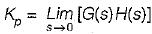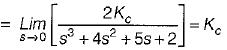∴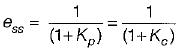(for a unit step input)
Given, ess = less than 2% of input
i.e. ess < 0.02
Now, characteristic equation is:
1 + G(s)H(s) = 0
or, s3 + 4s2 + 5s + 2 + 2Kc = 0
Routh’s array is: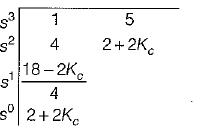For stability of given system,
2 + 2Kc > 0 or Kc > -1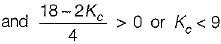or Kc < 9
Hence, -1 < Kc < 9 (for stability) ....... (1)
Now,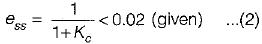From equations (1) and (2), we conclude that the minimum value of steady state error = 0.1 when Kc = 9.
Also, for all value of Kc between -1 and 9, value of ess will be more than 0.02 i.e. more than 2% of input.
Therefore, there is no such value of Kfor given system i.e. can not be determined.

QUESTION: 15

Match List-I (Controllers) with List-II (Transfer functions) and select the correct answer using the codes given below the lists: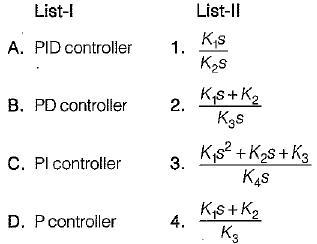Codes:
A B C D
(a) 2 3 4  1
(b) 3 4 1  2
(c) 3 4  2 1
(d) 3 1 4  2

Solution:
QUESTION: 16

The transfer function of a system is given as: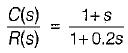It represents a

Solution:

Given,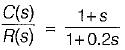The pole-zero plot is shown below.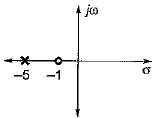Since zero is near to origin, therefore it represents a lead network.

QUESTION: 17

Integral feedback control

Solution:
QUESTION: 18

The industrial controller having the best steady state accuracy is

Solution:
QUESTION: 19

The transfer function of a phase lead controller is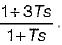The maximum value of phase shift provided by this controller is

Solution:

Given,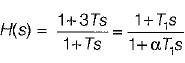Here, T1 = 3T and αT1 = T
∴ α = T/T= T/3T = 1/3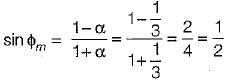or,  ϕm = 30° = maximum phase shift

QUESTION: 20

With regard to the filtering property, the lead compensator and the lag compensators are respectively

Solution: